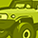# MATH overflow handlingNot applicable
Hi,

I'm trying the MATH overflow but the debugger never reaches th error handling section. What I made wrong?

`#include #include "XMC1300.h"/* variable initialization */uint32_t calculation_dividend;uint32_t calculation_divisor;uint32_t calculation_result;#define XMC_MATH_UNSIGNED_DIVISION                    ((uint32_t) 1 << MATH_DIVCON_USIGN_Pos)void XMC_MATH_DIV_UnsignedModNB_16(uint32_t dividend, uint32_t divisor){  MATH->DIVCON = XMC_MATH_UNSIGNED_DIVISION | 2 << MATH_DIVCON_DIVMODE_Pos;  MATH->DVD    = dividend;  MATH->DVS    = divisor;}int main(void) { /* configure DIV end-of-calculation interrupt */ XMC_MATH_EnableEvent(XMC_MATH_EVENT_DIV_END_OF_CALC);  XMC_MATH_EnableEvent(XMC_MATH_EVENT_DIV_ERROR);  NVIC_SetPriority(MATH0_0_IRQn,3); NVIC_EnableIRQ(MATH0_0_IRQn);  calculation_dividend = 0x8000; calculation_divisor = 0xFFFF;  XMC_MATH_DIV_UnsignedModNB_16(calculation_dividend,calculation_divisor); while(XMC_MATH_DIV_IsBusy()); for (int i=0; i<100; i++) ;  while (1) ;  return 1;}/* MATH Interrupt Handler */void MATH0_0_IRQHandler(void){ if (XMC_MATH_GetEventStatus(XMC_MATH_EVENT_DIV_ERROR)) {  XMC_MATH_ClearEvent(XMC_MATH_EVENT_DIV_ERROR);  calculation_result = 0;  } else {  XMC_MATH_ClearEvent(XMC_MATH_EVENT_DIV_END_OF_CALC);  calculation_result = XMC_MATH_DIV_GetUnsignedModResult(); }}`
3 Replies

# Re: MATH overflow handlingEmployee
EmployeeHello,

The overflow error detection feature is applicable only in the XMC1300 AB device and onwards.

Can you kindly check if you are using an AB device?
This can be done by simply reading the uppermost nibble of the SCU register DBGROMID:
- The value '1' indicates AA step
- The value '2' indicates AB step

Regards,
Min Wei

# Re: MATH overflow handlingNot applicable
DBGROMID.VERSION=2 on my eval board.

I found an another bug also: CGATSTAT0 default is 0x6FF (and not 0x7FF) because MATH enabled by default.

# Re: MATH overflow handlingEmployee
EmployeeThanks for checking the device version.

I believe the problem is because your code is using unsigned division mode whereas the overflow condition is applicable only for signed division mode.
Reason is the following:
- with signed numbers, the range is -32768 to 32767
- overflow condition occurs if -32768 (0x8000) is divided by -1 (0xFFFF) since the result would have been +32768.
- with unsigned division mode, 32768 divided by 65535 (0xFFFF) gives quotient of 0 and remainder of 32768. This is not considered as an overflow condition.

I can't explain the discrepancy between the actual and specified CGATSTAT0 register value though.
I will feedback to the team accordingly.

Regards,
Min Wei